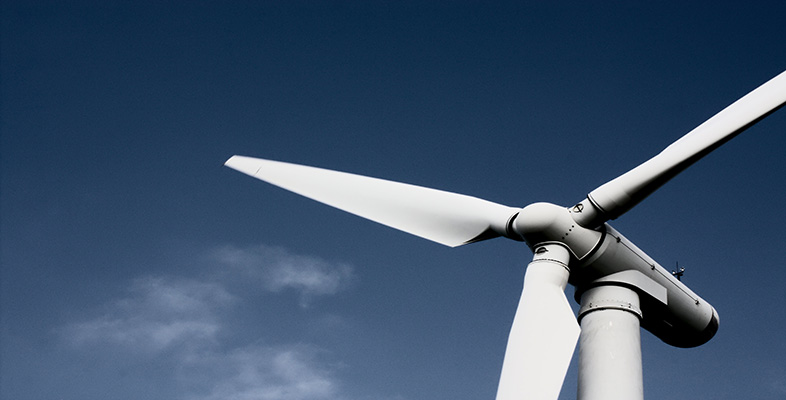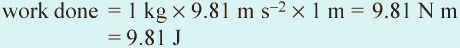An introduction to energy resources

Start this free course now. Just create an account and sign in. Enrol and complete the course for a free statement of participation or digital badge if available.

Free course

# 2.1 Some basic concepts

Energy is defined as the capacity to do work, and work is even more precisely defined as a force acting on an object that causes its displacement, and is calculated from force × distance. Work is therefore the foundation for scientific study of motion and change.

The unit of work is the same as that for energy: the joule (J). Yet a joule, unlike the units of mass, length or time, is not a fundamental unit. Working out the joule in fundamental units takes us to the root of the physics involved. The definition of work (force × distance) shows that one joule is actually one newton metre (N m).

Force is mass × acceleration, so one newton is the force that gives a mass of one kilogram (kg) an acceleration of one metre per second per second (m s−2), and is therefore equivalent to 1 kg m s−2.

However, work is not just mechanical movement, such as moving sacks of flour around or turning a wheel. It is also involved in heating a substance (vibrating its molecules), changing its state (melting and boiling) and compressing it, along with many other phenomena.

As an example of the connection between work and energy consider the energy bound up with a photon of light that strikes the photoelectric cell in a solarpowered pocket calculator. Some of that photon's energy becomes an electrical current that contributes to the calculator's microchip executing a calculation. However, most of the original light energy goes to heating up the cell and the liquid crystal display that shows the answer, and there might even be a beep of sound when the calculation is complete. During this process a tiny amount of work is done, but the form of the energy has changed, from the otherwise limitless potential of light to travel an infinite distance through a vacuum, never losing its 'capacity to do work', to the motion of particles of matter. The last change is eventually expressed by a minuscule rise in temperature in and around the calculator, which ultimately dissipates to help heat up the rest of the Universe! Energy can neither be created or destroyed (the Law of Conservation of Energy) so the energy of the photon doesn't disappear but is spread far and wide. Although eternal, in practice it is beyond recovery: useful energy in a high grade form is degraded through the material work that it has done.

Energy takes many natural forms: light, heat, sound, mechanical movement (kinetic energy), that gained by position in a gravitational field (potential energy), the movement of electrons (electricity), chemical energy, that released through Einstein's famous matter-energy conversion E = mc2 (nuclear energy) and a great many more. In this book we deal mainly with the forms of energy that are available at sufficiently high grade to be able to do useful work. An implicit theme is that all energy sources are themselves products of work done naturally:

• Chemical energy — 'fossil fuels', such as coal and petroleum, and 'biofuels', such as wood, are the products of conversion of solar energy into the energy of chemical combination through photosynthesis by plants;

• Nuclear energy — nuclear fuels are heavy radioactive isotopes produced by thermonuclear fusion in long-dead stars that became supernovae;

• Geothermal energy comes from heat produced by the natural decay of radioactive isotopes distributed at very low concentrations in the Earth;

• Solar energy is emitted by thermonuclear fusion within the Sun;

• Tidal energy is essentially the work done by the gravitational fields of the Moon and Sun on the oceans;

• Wind and wave energy are solar energy converted into work done by the Earth's atmosphere and oceans;

• Hydro energy relies on work done by solar energy in evaporating water that falls as rain or snow at high topographic elevations, which in turn has gravitational potential energy.

Every application that 'uses' energy is in fact converting one form of energy into other forms. Some of this energy conversion is useful, some is not. A kettle boils water, but it may also 'sing' (sound energy) and heat up itself. A hydroelectric power station turns the kinetic energy of moving water into electricity through generators, but at the same time friction between the moving parts of the generators produces heat and sound. The heat and sound are degraded and less useful forms of energy.

## Question 1

Which energy changes take place when an electric kettle boils water?

Electrical energy is first converted into heat energy as it increases the vibrational movement of atoms in the metal heating element. Heat is conducted through the element into the water, making the water molecules vibrate more, thereby raising its temperature. Finally, heat energy converts water into steam.

The point to be grasped here is that changes of energy from one form to another are commonplace in everyday life.

Power is the rate at which energy is delivered or work is done. It is worth noting the difference between power and energy: energy is an amount of work done over an indefinite time interval and power is the rate at which energy is converted, i.e. the amount used or made available per second. The units for measuring energy and power are summarised in Box 1.1 below.

## Box 1.1 Units of energy and power

All forms of energy are measured in the same unit, the joule (J). A joule is the same amount of energy irrespective of which form (light, sound, electrical, and so on) that energy takes.

Recalling the earlier definitions in this section, when 1 kg falls 1 m at the acceleration due to gravity at the Earth's surface (9.81 m s−2), the work done (force × distance) is mass in kg × acceleration due to gravity in m s−2 × height in m, i.e.:So a joule is equivalent to the work done when a mass of (1/9.81) kg or 0.102 kg falls through a metre: a mass about the size of the apple reputed to have fallen on Isaac Newton's head to inspire his theory of gravity! A joule is a small amount of energy so when considering national or international energy use, large multiples of the joule are needed, and these are named in the metric (SI) system using standard prefixes (Table 1.1).

### Table 1.1 Units of energy and power. In other sections you will also find the milliwatt (mW or 10−3 watts) used.

EnergyNumber in Powers of tenPower
UnitAbbreviationUnitAbbreviation
kilojoulekJ103kilowattkW
megajouleMJ106megawattMW
gigajouleGJ109gigawattGW
terajouleTJ1012terawattTW
exajouleEJ1018exawattEW

There is another energy unit that will be used occasionally in this book, the tonne oil equivalent (toe). This is often used by energy statisticians as a convenient means of comparing amounts of energy available from fuels other than oil. One toe is the chemical energy contained in one tonne of oil, and equates approximately to 4.19 × 1010 J (i.e. 41.9 GJ).

Being a rate, power is measured in joules converted per second (J s−1), called watts (W). One watt is equivalent to one joule per second. Note that if you are hit on the head by a falling apple, even though its energy is only about 1 J, the impact is almost instantaneous, so its power is high. Just as a joule is a small unit of energy, so a watt is a small unit of power. Larger quantities of power are quoted using the same prefixes as energy, as given in Table 1.1.

An electric supply of 1 kW will run a microwave oven, or around 10 light bulbs. Working really hard, the human body can deliver about 1 kW for a very short period of time.

Every three months or so most people in the UK receive an electricity bill, which states the number of 'Units' of electricity they have used, as measured by their electricity meter. The 'Units' quoted are kilowatt hours (kW h). But what, in purely physical terms, does 1 kW h represent? One kilowatt hour is the energy delivered at a power of 1 kW for one hour. This is equivalent to 1 kJ per second for an hour, i.e. 3600 kJ.

In practical terms, you might think that one kW h was a measure of all the work done around your home by various appliances in an hour. You would be very disappointed, because not one of them is perfectly efficient. In fact over 70% of each 'Unit' would ultimately have been lost to heating the rest of the Universe without doing any useful work. As you will see below, generating and then transmitting the electrical energy to your home will have been inefficient too, so a high proportion of your bill had been spent on work that was of no practical use to you.

When we convert energy from one form to another, the output that is useful to us is never as much as the energy input. The ratio of the useful output to the input (i.e. the ratio of useful work done to the energy supplied) is called the energy efficiency of the process and is usually expressed as a percentage. Every natural process involves a change in grade of energy through the work that it does, but one joule always ends up as one joule, because of the Law of Conservation of Energy. Ignoring this transformation in grade, energy conversion could be said to be 100% efficient. But that misses the point. A perfect energychanging machine would get out as much useful energy as was put in, so it would be 100% efficient, but such a machine does not and probably cannot exist.

Efficiency can be as high as 90% in a water turbine, but only around 35-40% in a coal-fired power station. The energy delivered to do useful work is considerably less than the primary energy consumed in electricity generation.

S278_2